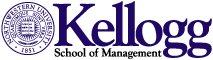Data for Testing Standard Error Estimation Programs

To test the different programs, I created a test data set. The data set contains four variables: a firm identifier (firmid), a time variable (year), the independent variable (x), and the dependent variable (y). The residual and the independent variable both contain a firm effect, but no year effect. Thus the standard errors clustered by firm are different from the OLS standard errors (and the standard errors clustered by firm and year are different than the standard errors clustered by year). I have posted this data set as a text file and as a Stata data set. The results of running the OLS regression with OLS standard errors, White standard errors and clustered standard errors – as well as Fama-MacBeth coefficients and standard errors are reported below.

### OLS Coefficients and Standard Errors

 Variable Coefficient Standard Error T-statistic Constant 0.0297 0.0284 1.05 X 1.0348 0.0286 36.20 R2 = 0.2078

### OLS Coefficients and White Standard Errors

 Variable Coefficient Standard Error T-statistic Constant 0.0297 0.0284 1.05 X 1.0348 0.0284 36.44 R2 = 0.2078

### OLS Coefficients and Standard Errors Clustered by Firm

 Variable Coefficient Standard Error T-statistic Constant 0.0297 0.0670 0.44 X 1.0348 0.0506 20.45 R2 = 0.2078

### OLS Coefficients and Standard Errors Clustered by Year

 Variable Coefficient Standard Error T-statistic Constant 0.0297 0.0234 1.27 X 1.0348 0.0334 30.99 R2 = 0.2078

### OLS Coefficients and Standard Errors Clustered by Firm and Year

 Variable Coefficient Standard Error T-statistic Constant 0.0297 0.0651 0.46 X 1.0348 0.0536 19.32 R2 = 0.2078

### Fama-MacBeth Coefficients and Standard Errors

 Variable Coefficient Standard Error T-statistic Constant 0.0313 0.0234 1.34 X 1.0356 0.0333 31.06 R2 = 0.2078

## Clustering in Multiple Dimensions in SAS

In SAS you can specify multiple variables in the cluster statement. For example, you could put both firm and year as the cluster variables. Using the test data set, I ran the regression in SAS and put both the firm identifier (firmid) and the time identifier (year) in the cluster statement. The SAS commands are:

proc surveyreg data=mydata;
cluster firmid year;
model y  = x ;

The results are:

 Variable Coefficient Standard Error T-statistic Constant 0.0297 0.0284 1.05 X 1.0348 0.0284 36.44 R2 = 0.2078

These are White standard errors, not standard errors clustered by both firm and time. To see this, compare these results to the results above for White standard errors and standard errors clustered by firm and year. The reason is when you tell SAS to cluster by firmid and year it allows observations with the same firmid and and the same year to be correlated. Since there is only one observation for each firm year in the sample, this assumes all residuals are uncorrelated (SAS assumes there are 5,000 clusters). In my paper, in Thompson (2006) and in Cameron, Gelbach and Miller (2006),  when we discussed clustering by firm and year, this allows the residuals of observations from the same firm or the same year to be correlated.